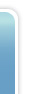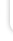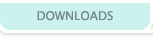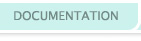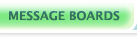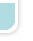Wraparound Maps: Done!
Displaying 1-1 of 1 total.
1
Please enter a numerical value for the importance of this sticky.
Enter 0 to unsticky.
 rafael_esperOk, as I've mentioned earlier, this was in my top 3 list of verge features I'd like to see. Well, the code below is in Java, but it's very close to the original CPP code. I've implemented this in my Java verion of Verge (I'm calling it JVerge), and it's almost ready for a first release (http://verge-rpg.com/forums/verge-developers-forum/jverge/) Here it goes: In the Map, add two variables to control if the map is wrapable (horizontal-edges and vertical-edges): ``` public boolean horizontalWrapable = false; // new code public boolean verticalWrapable = false; // new code ``` Of course, you need to set these variables at some point for your World maps, like: ``` current_map.horizontalWrapable = current_map.verticalWrapable = true; ``` In the Render method, if the map is wrapable, don't limit the xwin and ywin: ``` case 1: if( myself != null ) { xwin = (myself.getx() + myself.chr.hw/2) - (screen.width / 2); ywin = (myself.gety() + myself.chr.hh/2) - (screen.height / 2); } else { xwin=0; ywin=0; } if(!current_map.horizontalWrapable) { // new code if (xwin + screen.width >= rmap) xwin = rmap - screen.width; if (xwin < 0) xwin = 0; } if(!current_map.verticalWrapable) { // new code if (ywin + screen.height >= dmap) ywin = dmap - screen.height; if (ywin < 0) ywin = 0; } break; ``` In the method obstructpixel of the Map class, the edges aren't obstructable anymore for wrapable maps. My (messy) code is like this: ``` public boolean obstructpixel(int x, int y) { // modified by rbp if (!horizontalWrapable && (x < 0 || (x >> 4) >= getWidth())) return true; if (!verticalWrapable && (y < 0 || (y >> 4) >= getHeight())) return true; if(horizontalWrapable && x < 0) x+= (getWidth() << 4); if(horizontalWrapable && (x >> 4) >= getWidth()) x-= (getWidth() << 4); if(verticalWrapable && y < 0) y+= (getHeight() << 4); if(verticalWrapable && (y >> 4) >= getHeight()) y-= (getHeight() << 4); int t = obsLayer[((y >> 4) * getWidth()) + (x >> 4)]; if (tileset.GetObs(t, x & 15, y & 15) == 0) return false; return true; } ``` In the same class, in the BlitLayer method, now you want to render the beginning of the map when you're close to its end, and vice-versa: Original code was something like: c = layer.GetTile(xtc + x, ytc + y); Now you need to change it to: ``` if(horizontalWrapable && verticalWrapable) // New code c = layer.GetTile((xtc + x+getWidth())%(getWidth()), (ytc + y+getHeight())%(getHeight())); else if(!horizontalWrapable && verticalWrapable) c = layer.GetTile((xtc + x), (ytc + y+getHeight())%(getHeight())); else if(horizontalWrapable && !verticalWrapable) c = layer.GetTile((xtc + x+getWidth())%(getWidth()), (ytc + y)); else if(!horizontalWrapable && !verticalWrapable) c = layer.GetTile(xtc + x, ytc + y); ``` In the Entity class, the getx() and gey() methods need to "teleport" the entity to the beginning of the map, if they go past its end. Also, we need to adapt the waypoint, so the entity won't walk backwards when it reachs the end of the map (because the entity X is 100, and the waypointX 116 can be converted to 16, in a 100x100 map): ``` public int getx() { if(current_map.horizontalWrapable && (x/65536 > current_map.getWidth()<<4 || x < 0)) { x = (x + (current_map.getWidth()<<20)) % (current_map.getWidth()<<20); waypointx = (waypointx + (current_map.getWidth()<<4)) % (current_map.getWidth()<<4); } return x/65536; } public int gety() { if(current_map.verticalWrapable && (y/65536 > current_map.getHeight()<<4 || y < 0)) { y = (y + (current_map.getHeight()<<20)) % (current_map.getHeight()<<20); waypointy = (waypointy + (current_map.getHeight()<<4)) % (current_map.getHeight()<<4); } return y/65536; } ``` Remember to make the class more OO, and all instances do entity.x and entity.y are converted to entity.getx() and entity.gety(). Finally, the draw method of entity needs to render the entities that are on the other side of the map: Instead of ``` int zx = getx() - xwin, zy = gety() - ywin; ``` Change it to: ``` int zx = (getx() - xwin + (current_map.getWidth()<<4)) % (current_map.getWidth()<<4), zy = (gety() - ywin + ((current_map.getHeight()<<4))) % (current_map.getHeight()<<4); ``` And that's it. It can be easily adapted to CPP code. Wraparound maps in Verge! Tested with my old Phantasy Star maps. Regards, Rafael, the Esper Posted on 2012-01-22 15:34:32 (last edited on 2012-01-22 15:35:29)

Displaying 1-1 of 1 total.
1I Like Ham.(by ThinIce on 2018-08-17)Steamworks Integration(by Eldritch05 on 2018-08-16)Entity Issues(by Rysen on 2018-06-05)Epic of Serinor: Dawnshadow(by Rysen on 2018-05-13)More Posts...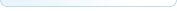Ben McGraw's lovingly crafted this website from scratch for years.
It's a lot prettier this go around because of Jon Wofford.
Verge-rpg.com is a member of the lunarnet irc network, and would like to take this opportunity to remind you that regardless how babies taste, it is wrong to eat them.